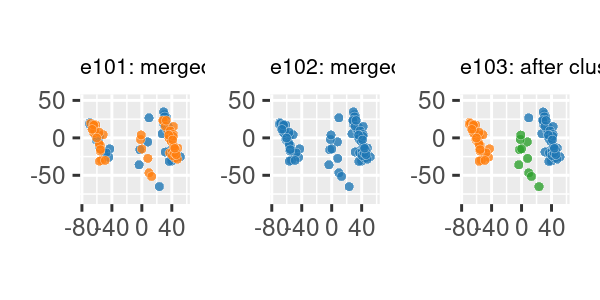# Merging and hierarchical cluster examples

This section contains several examples on how to merge cluster data, either generated with clugenr or from other sources. Although it is possible to merge data in any dimension, these examples will focus on merging 2D data. Therefore, we’ll use the same plot_examples_2d() function used for the vignette("examples2d"), requiring the following setup code:

# Load the clugenr library
library(clugenr)

# Load functions for plotting examples
source("plot_examples_2d.R", local = knitr::knit_global())

# Keep examples reproducible in newer R versions
RNGversion("3.6.0")

## Merging two data sets generated with clugen()

seed1 <- 4444
seed2 <- 5555
e090 <- clugen(2, 5, 1000, c(1, 1), pi / 12, c(20, 20), 14, 1.2, 1.5, seed = seed1,
proj_dist_fn = "unif", point_dist_fn = "n")
e091 <- clugen(2, 3, 1500, c(1, 0), 0.05, c(20, 20), 0, 0, 4, seed = seed2,
point_dist_fn = "n", cluster_offset = c(20, 0))
e092 <- clumerge(e090, e091)
plot_examples_2d(list(e = e090, t = "e090: data set 1"),
list(e = e091, t = "e091: data set 2"),
list(e = e092, t = "e092: merged data sets"))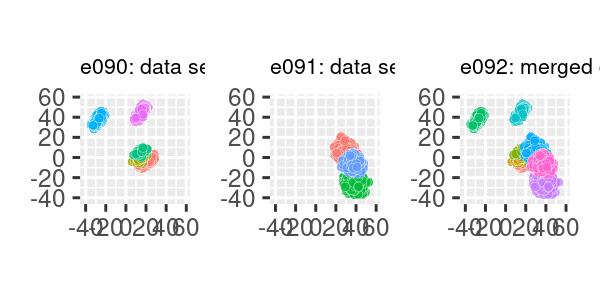In the previous example, clusters from individual data sets remain as separate clusters in the merged data set. It’s also possible to maintain the original cluster labels by setting the clusters_field parameter to NA:

e093 <- clumerge(e090, e091, clusters_field = NA)
plot_examples_2d(list(e = e090, t = "e090: data set 1"),
list(e = e091, t = "e091: data set 2"),
list(e = e093, t = "e093: merged data sets"),
palette = "set2")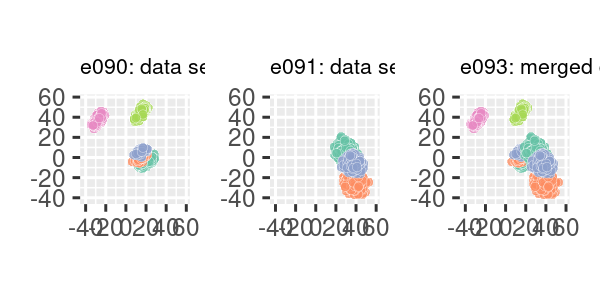## Adding noise to a clugen()-generated data set

set.seed(333)

e094 <- list(points = matrix(120 * runif(2 * 500) - 60, ncol = 2),
clusters = factor(rep(9L, 500)))     # Assign noise to cluster 9
e095 <- clumerge(e094, e092, clusters_field = NA) # and keep it 9 when merging
plot_examples_2d(list(e = e092, t = "e092: original merged data sets"),
list(e = e094, t = "e094: random uniform noise"),
list(e = e095, t = "e095: data sets with noise"),
palette = "set2", pmargin = -0.1)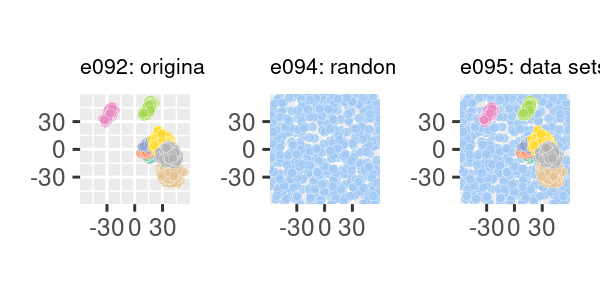## Merging with data not generated with clugen()

Data generated with clugen() can be merged with other data sets, for example as a way of augmenting them. In this example we perform stats::prcomp() to the datasets::swiss dataset to reduce its 6 variables to 2. As there are only 47 observations in this dataset, we’ll use clugen() to generate very similar clusters and then use clumerge() to create an augmented dataset:

set.seed(888)
spc <- prcomp(swiss)
e096 <- list(points = spc$x[, 1:2], clusters = factor(rep(4L, dim(spc$x))))
e097 <- clugen(2, 3, 50, c(-0.3, 1), 0., c(0, 0), 0, 0, 3,
proj_dist_fn = "unif", clusizes_fn = c(20, 6, 25),
clucenters_fn = matrix(c(-58, -5, 10, -40, 38, 0),
byrow = TRUE, ncol = 2),
angle_deltas_fn = c(0.02, -0.03, 0.1),
llengths_fn = c(55, 100, 60))
e098 <- clumerge(e096, e097, clusters_field = NA)
plot_examples_2d(list(e = e096, t = "e096: swiss PCA"),
list(e = e097, t = "e097: clugen-generated"),
list(e = e098, t = "e098: merged"),
palette = "seaborn")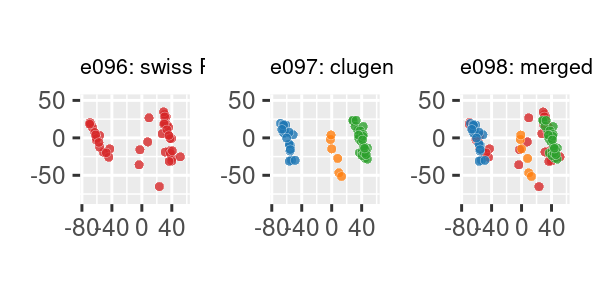We can also hierarchize clusters from different sources:

e099 <- append(e096, list(hclusters = factor(rep(1L, dim(spc$x))))) e100 <- append(e097, list(hclusters = factor(rep(2L, length(e097$clusters)))))
e101 <- clumerge(e099, e100, fields = "points", clusters_field = "hclusters")
plot_examples_2d(list(e = e099, t = "e099: swiss PCA (1 cluster)"),
list(e = e100, t = "e100: clugen-generated (1 cluster)"),
list(e = e101, t = "e101: merged (2 clusters)"),
clusters_field = "hclusters",
palette = "seaborn")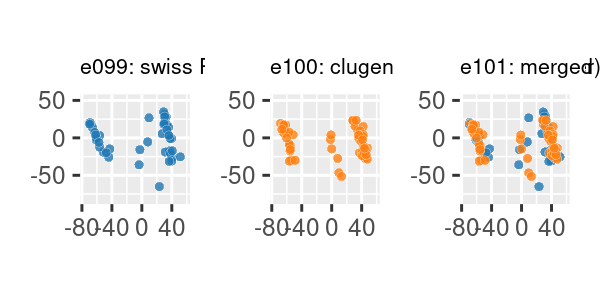Note that augmenting a dataset this way is probably not a statistically sound approach for most problems. In any case, if we perform clustering on the merged dataset and assume there are 3 clusters, results are interesting:

# For display purposes, assign all points in merged data to a single cluster
e102 <- list(points = e101$points, hclusters = factor(rep(1L, length(e101$hclusters))))

# Perform hierachical clustering with Manhattan distance, complete linkage and
# assuming 3 clusters
clusts <- cutree(hclust(dist(e101$points, method = "manhattan")), 3) # Create data set with merged points and new cluster labels e103 <- list(points = e101$points, hclusters = factor(clusts))
plot_examples_2d(list(e = e101, t = "e101: merged (2 clusters)"),
list(e = e102, t = "e102: merged (1 cluster)"),
list(e = e103, t = "e103: after clustering (3 clusters)"),
clusters_field = "hclusters",
palette = "seaborn")